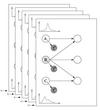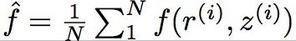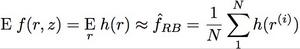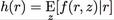## July 16, 2004

### What exactly is Rao-Blackwellisation?From Policy Recognition in the Abstract Hidden Markov Model:
Rao-Blackwellisation is a general technique for improving the accuracy of sampling methods by analytically marginalizing some variables and only sampling the remainder. In its simplest form, consider the problem of estimating the expectation E [f(x)], where x is a joint product of two variables r,z.
Using direct Monte-Carlo sampling, we obtain the estimator:Alternatively, a Rao-Blackwellised estimator can be derived by sampling only the variable r, with the other variable z, being integrated out analytically:where.
For our convenience, r will be referred to as the Rao-Blackwellising variable. The Rao-Blackwellised estimator \hat{f}_{RB} is generally more accurate than \hat{f} for the same number of samples N. Posted by djp3 at July 16, 2004 12:10 PM | TrackBack (0)
Comments
Post a comment